95%的用户最终下载了:

约有284939篇,以下是第1-10篇排序：相关性|最多阅读|最新上传只显示优质文档只显示可投资文档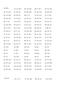52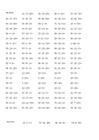[二年级数学]非常强大的小学100以内加减混合运算口算题大整理.doc
[二年级数学]非常强大的小学100以内加减混合运算口算题大整理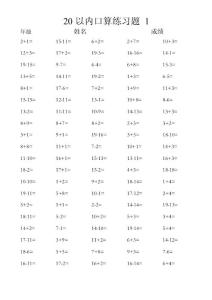53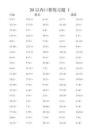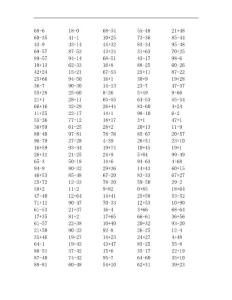57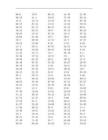• 玲玲 2014-01-22
• 热度:
• 1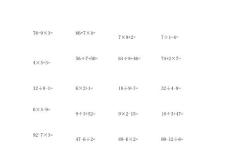64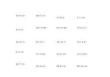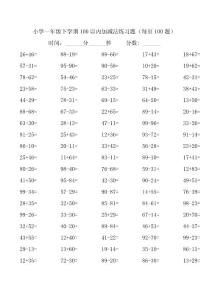34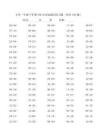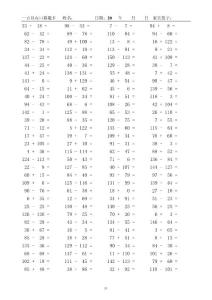50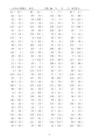• 萍儿 2011-09-28
• 热度:
• 8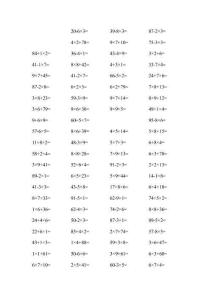160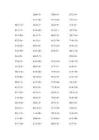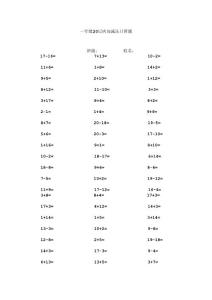55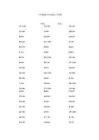73[一年级数学]一年级100以内加减法口算A4直接打印.doc
[一年级数学]一年级100以内加减法口算A4直接打印 日期 时间 分数 一年级 100 以内口算题 签字 20+5= 88-8= 86-77= 51+27= 13+74= 80+18= 93-33= 34+53= 72+23= 40-33= 76+14= 83-62= 69-8= 98-44= 64-32= 48-29= 39-36= 8+91= 89-53= 6+67= 55+5= 23-17= 43+42= 68+15= 64-4= 68-11= 10+15= 2+94= 1 87-72= 62+26= 75+24= 24+43= 74-55= 1+18= 100-57= 76-65= 75-14= 10+32= 62+32= 78-15= 50+16= 76+4= 日期 时间 分数 一年级 100 以内口算题 签字 65+9= 29+67= 39-23= 82+13= 93-38= 73+23= 95-26= 57+19= 39+9= 90-29= 89-42= 44-28= 8+10= 50-19= 21+5= 34-16= 95-83= 17+43= 91-37= 78-57= 68-44= 71-17= 9+5= 22+38= 27-14= 29+30= 83-31= 74-68= 2 100-56= 36+49= 45+13= 13+55= 59-54= 4-1= 84+1= 3+67= 54+46= 52-11= 33-9= 58-8= 12+7= 58+16= 日期 时间 分数 一年级 100 以内口算题 签字 18+56= 79-32= 20+26= 34-13= 27+34= 89-67= 5+48= 96-43= 92-63= 48-47= 53+22= 16+70= 46-29= 12+58= 27+70= 24+49= 45-43= 65-23= 100-28= 37+47= 42+2= 48-7= 17+1= 50+18= 63+10= 59-38= 23-6=..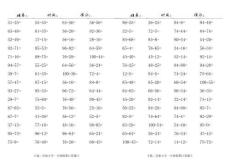61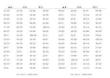[一年级数学]100以内加减法口算题A4.doc
[一年级数学]100以内加减法口算题A4 姓名： 51‒35= 65‒48= 52‒49= 92‒71= 71‒16= 94‒57= 39‒7= 57‒47= 93‒27= 29‒7= 87‒65= 67‒7= 37‒15= 95‒73= 75‒9= 时间： 55‒45= 81‒35= 17‒15= 95‒53= 89‒75= 55‒25= 61‒35= 97‒15= 95‒33= 75‒69= 55‒5= 41‒36= 77‒16= 96‒13= 76‒48= 84‒56= 36‒20= 56‒16= 96‒92= 76‒28= 64‒56= 100‒36= 36‒16= 96‒72= 76‒40= 76‒56= 36‒12= 48‒16= 96‒84= 76‒20= 得分： 56‒56= 92‒36= 28‒16= 64‒59= 100‒44= 36‒24= 72‒4= 84‒8= 64‒44= 80‒45= 25‒16= 52‒5= 88‒85= 65‒24= 60‒45= 姓名： 96‒25= 32‒5= 85‒68= 65‒4= 45‒40= 76‒25= 12‒5= 85‒48= 84‒65= 45‒20= 56‒25= 92‒5= 85‒28= 65‒64= 100‒45= 时间： 36‒25= 72‒5= 85‒8= 76‒65= 45‒12= 48‒25= 84‒5= 85‒20= 66‒56= 92‒14= 94‒28= 74‒64= 100‒54= 36‒34= 72‒14= 94‒8= 74‒44= 80‒54= 34‒16= 52‒14= 94‒88= 74‒24= 60‒54= 96‒34= 32‒14= 94‒68= 74‒4= 54‒40= 76‒34= 14‒12= 得分： 94‒48= 84‒74= 54‒20= 56‒34= 92‒14= 94‒28= 75‒64= 100‒55= 36‒35= 71‒15= 95‒7= 92‒29= 95‒9= 47‒43= 75‒72= 丰满二实验小学一年级假期口算题卡 丰满二实验小学一年级假期口算题卡 姓名： 67‒28= 74‒51= 82‒53= 63‒9= 27‒3= ..